STAT 330: 98-1

1. Sometimes people looked up F-critical points in order to carry out tests at specific levels as requested in the text. This is not wrong but also not necessary. If P < 0.01 then you can reject the null at the level 0.01. That's why you only need the P-value.
2. A P-value does not measure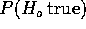. After all, you must assume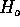is true to compute P. Instead it measures how often, when the null is true, you would expect to see a test statistic as extreme as the one you actually saw.
3. You need to interpret the output not just hand it in. Your conclusions must be about real world things not just ``I reject.''
4. In one question the Tukey intervals all include 0 but the F test of the hypothesis rejects the null. The interpretation is that while we are confident that not all the means are equal we aren't quite sure which ones differ from which; we are unable to name with adequate confidence a specific pair which differ.
5. If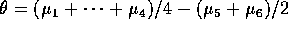then we estimateusing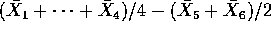. The variance of this is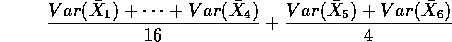which is simply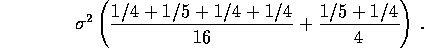Now plug in MSE for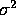and take a square root to get the estimated standard error of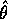and use the interval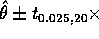(estimated standard error).

Richard Lockhart
Mon Mar 23 23:32:29 PST 1998